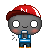部落格成立計數器  （文字）

<font color=#3366cc size=3>天使的禁忌第<script language="JavaScript">var now=new Date();var spday=new Date(2008,02-1,16);a=(now.getTime()-spday.getTime())/(24*60*60*1000);a=Math.ceil(a); document.write("<b>"+a+""); </script></font>

<font color=#3366cc size=3>活在世上<script language="JavaScript">var now=new Date();var spday=new Date(1995,09-1,11);a=(now.getTime()-spday.getTime())/(24*60*60*1000);a=Math.ceil(a); document.write("<b>"+a+""); </script></font>複製語法 Copy Here生日倒數 （文字）

<font color=#3366cc size=3>離13歲生日還有<script language="JavaScript">var now=new Date();var spday=new Date(2008,09-1,11);a=(spday.getTime()-now.getTime())/(24*60*60*1000);a=Math.ceil(a); document.write("<b>"+a+""); </script></font>複製語法 Copy Here每日問候語 （文字）

<script language="JavaScript">
<!--
document.write("<font size=3>")
now = new Date()
if (now.getDay() == 0)
document.write("星期日！享受個美好的一天！")
if (now.getDay() == 1)
document.write("星期一！呵~要開始工作囉！")
if (now.getDay() == 2)
document.write("星期二~呼！星期一終於過了！")
if (now.getDay() == 3)
document.write("星期三，要上哪玩ㄚ？")
if (now.getDay() == 4)
document.write("星期四，哈~明天就是星期五嘍！")
if (now.getDay() == 5)
document.write("哇！終於盼到星期五了！")
if (now.getDay() == 6)
document.write("星期六啦~祝您假期愉快！")
document.write("</font>")
-->
</script>小小的叮嚀：  記住喔，以上的語法都可以放在網誌描述或是自定欄位，但無名部落格支部支援我就不太了解了。

--------------------------------------------------